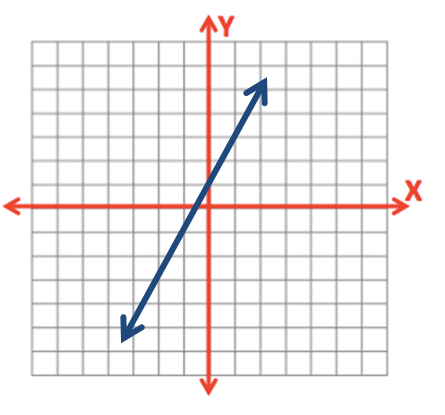# 0.1.3 - Basic Linear Equations

You may recall from an algebra class that the formula for a straight line is $$y=mx+b$$ where $$m$$ is the slope and $$b$$ is the y-intercept. The x-axis is the horizontal axis and the y-axis is the vertical axis.The y-intercept is where the line crosses the y-axis.  The slope is a measure of change in y over change in x, sometimes written as $$\frac{\Delta y}{\Delta x}$$ where $$\Delta$$ ("delta") means change.

## Example Section

$$y=2x+1$$

Here, the y-intercept is 1 and the slope is 2.  This line will cross the y-axis at the point (0, 1). From there, for every one increase in $$x$$, $$y$$ will increase by 2. In other words, for every one unit we move to the right, we will move up 2.In statistics, the y-intercept is typically written before the slope. The equation for the line above would be written as $$y=1+2x$$.

Different notation may also be used:

$$\widehat y = a + bx$$, where $$\widehat y$$ is the predicted value of y, $$a$$ is the y-intercept and $$b$$ is the slope.

$$\widehat y = b_0 + b_1 x$$, where $$\widehat y$$ is the predicted value of y, $$b_0$$ is the y-intercept, and $$b_1$$ is the slope.